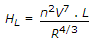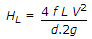# Civil Engineering - Water Supply Engineering

### Exercise :: Water Supply Engineering - Section 4

26.

Normal values of overflow rate for plain sedimentation tank using coagulants, is

 A. 750 to 1000 litres/hr/m2 B. 1000 to 1250 litres/hr/m2 C. 1250 to 1500 litres/hr/m2 D. 1500 litres/hr/m2.

Explanation:

No answer description available for this question. Let us discuss.

27.

The formula Q = P - K[1.8T + 32] in which Q is runoff, P is annual rain fall in cm, T is mean annual temperature in centigrades and K is a constant, is known

 A. Justin's formula B. Khosla's formula C. English formula D. Vermule's formula.

Explanation:

No answer description available for this question. Let us discuss.

28.

The formula for pipe flows, suggested by, Hazen-William is

 A.B. V = 0.85 CH R0.63 . S0.54 C.D. None of these.

Explanation:

No answer description available for this question. Let us discuss.

29.

While selecting the location of an intake for collecting surface water, the factor considered, is

 A. The intake point should be near as far as possible to the treatment plant B. The intake point should be in purer zone of the water source C. The intake point should be upstream of the point of disposal of waste water D. The intake point in meandering rivers should be on concave banks E. All the above;

Explanation:

No answer description available for this question. Let us discuss.

30.

Aqueducts are generally designed

 A. circular B. rectangular C. horse shoe section D. all the above.